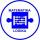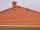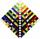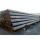# AS sequence

In an arithmetic sequence is given the difference d = -3 and a71 = 455.

a) Determine the value of a62
b) Determine the sum of 71 members.

Result

a62 =  482
s71 =  39760

#### Solution:Leave us a comment of example and its solution (i.e. if it is still somewhat unclear...):

Showing 0 comments:Be the first to comment!## Next similar examples:

1. AP - basicsDetermine first member and differentiate of the the following sequence: a3-a5=24 a4-2a5=61
2. AP - simpleDetermine the first nine elements of sequence if a10 = -1 and d = 4
3. Sequence 3Write the first 5 members of an arithmetic sequence: a4=-35, a11=-105.
4. SequenceBetween numbers 1 and 53 insert n members of the arithmetic sequence that its sum is 702.
5. SequenceWrite the first 7 members of an arithmetic sequence: a1=-3, d=6.
6. SequenceWrite the first 6 members of these sequence: a1 = 5 a2 = 7 an+2 = an+1 +2 an
7. AS - sequenceWhat are the first ten members of the sequence if a11=22, d=2.
8. Sequence 2Write the first 5 members of an arithmetic sequence a11=-14, d=-1
9. Nineteenth memberFind the nineteenth member of the arithmetic sequence: a1=33 d=5 find a19
10. TreesA certain species of tree grows an average of 0.5 cm per week. Write an equation for the sequence that represents the weekly height of this tree in centimeters if the measurements begin when the tree is 200 centimeters tall.
11. Third memberDetermine the third member of the AP if a4=93, d=7.5.
12. Sum of membersWhat is the sum of the first two members of the aritmetic progression if d = -4.3 and a3 = 7.5?
13. SeatsSeats in the sport hall are organized so that each subsequent row has five more seats. First has 10 seats. How many seats are: a) in the eighth row b) in the eighteenth row
14. RoofTiles are stacked in rows on the trapezoidal shaped roof. At the ridge is 15 tiles and each subsequent row has one more tile than in the previous row. How many tiled is covered roof if lowermost row has 37 tiles?
15. SeriesYour task is express the sum of the following arithmetic series for n = 14: S(n) = 11 + 13 + 15 + 17 + ... + 2n+9 + 2n+11
16. TubesIron tubes in the warehouse are stored in layers so that each tube top layer fit into the gaps of the lower layer. How many layers are needed to deposit 100 tubes if top layer has 9 tubes? How many tubes are in bottom layer of tubes?
17. Theorem proveWe want to prove the sentense: If the natural number n is divisible by six, then n is divisible by three. From what assumption we started?